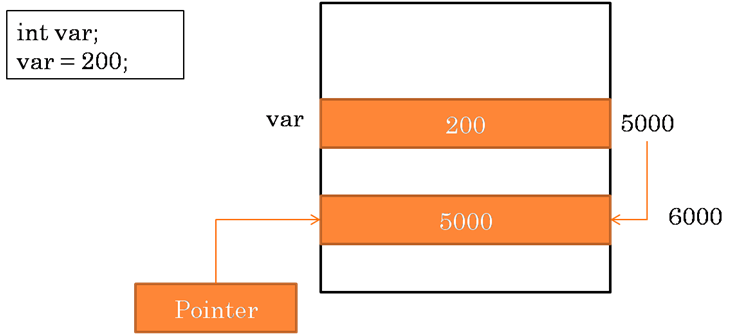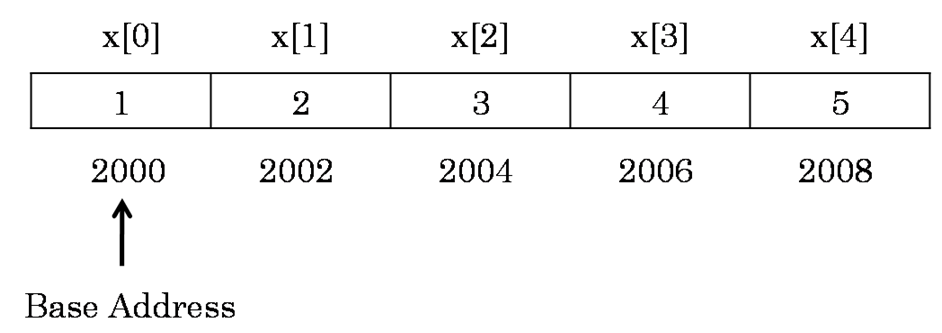My Blog
Articles related to programming, computer science, technology and research.

23/04/2016 Categories: C Programming. Comments Off on Pointers in C

A pointer is a derived data type in C. It is built from one of the fundamental data types available in C.

Pointers contain memory addresses as their values. Since these memory addresses are the locations in the computer memory where program instructions and data are stored, pointers can be used to access and manipulate data stored in the memory.

## Advantages of Pointers

Pointers are used frequently in C, as they offer a number of benefits to the programmers. They include the following:

1. Pointers are more efficient in handling arrays and data tables.
2. Pointers can be used to return multiple values from a function via function arguments.
3. Pointers allow passing a function as argument to other functions.
4. The use of pointer arrays to character strings results in saving of data storage space in memory.
5. Pointers allow C to support dynamic memory management.
6. Pointers provide an efficient way for manipulating dynamic data structures such as structures, linked lists, queues, stacks and trees.
7. Pointers increase the execution speed and thus reduce the program execution time.

## Understanding Pointers

The computer’s memory is a sequential collection of storage cells. Each cell, commonly known as a byte, has a number called address associated with it.

Typically, the addresses are numbered consecutively, starting from zero. The last address depends on the memory size. A computer system having 64k memory will have its last address as 65,535.

Whenever we declare a variable in our programs, the system allocates somewhere in the memory, an appropriate location to hold the value of the variable. This location will have its own address number. Consider the following example:The above statement int var creates a location in the memory to hold integer value. That location will have an address for example assume it is 5000. The statement var = 200 stores the value 200 at the location whose address is 5000. So, in our example, var is the variable name, 200 is the value stored in var and 5000 is the address of the memory location containing the variable var.

So, we can access the value 200 either by using the variable name var or by using the address of the memory location which is 5000.

We can access using the second method by storing the address in another variable. This concept of storing memory address in a variable and accessing the value available at that address is known as a pointer variable.

Since the pointer is also variable, it will also have a memory address just like any other variable.

#### Pointer Constants

The memory addresses within a computer are referred to as pointer constants.

#### Pointer Values

We cannot assign the memory addresses directly to a variable. We can only get the address of a variable by using the address operator (&). The value thus obtained is known as pointer value.

#### Pointer Variables

Once we obtain the pointer value, we can store it in a variable. Such variable which stores the memory address is known as a pointer variable.

### Accessing the Address of a Variable

The actual location of a variable in memory is system dependent. A programmer cannot know the address of a variable immediately.

We can retrieve the address of a variable by using the address of (&) operator. Let’s look at the following example:

In the above example, let the variable a is stored at memory address 5000. This can be retrieved by using the address of operator as &a. So the value stored in variable p is 5000 which is the memory address of variable a. So, both the variable a, and p point to the same memory location.

### Declaring and Initializing Pointer Variables

#### Declaration

In C, every variable must be declared for its type. Since pointer variables contain addresses that belong to a specific data type, they must be declared as pointers before we use them.

The syntax for declaring a pointer is as shown below:

This tells the compiler three things about the variable pointer-name. They are:

1. The asterisk (*) tells that the variable pointer-name is a pointer variable.
2. pointer-name needs a memory location.
3. pointer-name points to a variable of type datatype.

Consider the following example for declaring pointers:

#### Initialization

After declaring a pointer variable we can store the memory address into it. This process is known as initialization. All uninitialized pointers will have some unknown values that will be interpreted as memory addresses. Since compilers do not detect these errors, the programs with uninitialized pointers will produce erroneous results.

Once the pointer variable has been declared, we can use the assignment operator to initialize the variable. Consider the following example:

In the above example, we are assigning the address of variable var to the pointer variable p by using the assignment operator. We can also combine the declaration and initialization into a single line as shown below:

### Accessing a Variable Using Pointer

After declaring and initializing a pointer variable, we can access the value of the variable pointed by the pointer variable using the * operator. The * operator is also known as indirection operator or dereferencing operator. Consider the following example:

In the above example, we are printing the value of the variable var by using the pointer variable p along with the * operator.

## Pointers and Arrays

When an array is declared in a program, the compiler allocates a base address and sufficient amount of storage to contain all the elements of the array in contiguous (continuous) memory locations.

The base address is the location of the first element (index 0) of the array. The compiler also defines the array name as a constant pointer to the first element. Suppose array x is declared as shown below:

Suppose the array is allocated memory as shown below:The name x is defined as a constant pointer pointing to the first element, x and therefore the value of x is 2000, the location where x is stored.

If we declare p as an integer pointer, then we can make the pointer p point to the array x by the following assignment:

In a one dimensional array the address of element n can be calculated by using the below expression:

address = base address + (index * scale factor)

In a two dimensional array with m rows and n columns the address of an element can be calculated as shown below:

address = base address + [(i * n + j) * scale factor]

Where i represents ith row and j represents jth column.

To be continued…

Scroll Up